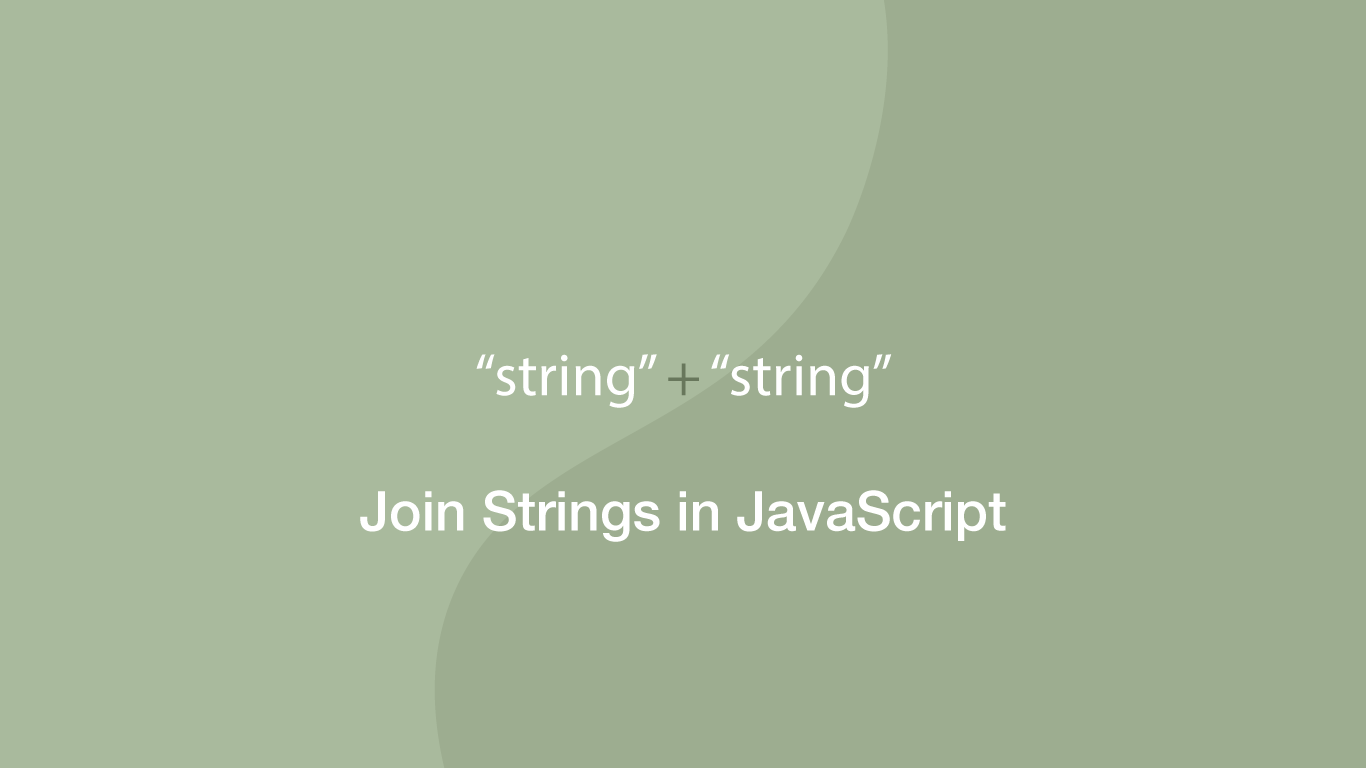# How to Join Two Strings in JavaScriptJoining strings in JavaScript is a common task, one example of this might be to produce a formatted output for the user containing all the relevant information from multiple strings. This is formally known as concatenation and in this tutorial, we will explore some of the different ways we can combine two or more strings in JavaScript.

## Using the + Operator

The easiest way to concatenate strings is to use the `+` (plus) operator. To use it, define a new variable and pass in each string separated by a `+` (plus).

``````str1 = 'hello';
str2 = 'john ';

output = str1 + ' ' + str2;

console.log(output);
``````
``````hello john
``````

In the example above we are also adding a space character string between the other strings.

Another way to approach this is to use the `+=` (plus and equals) operator, which is a shorter way to add one string to another string.

``````str = 'hello';
str += ' ';
str += 'john ';

console.log(str);
``````
``````hello john
``````

## Using the .concat() Method

JavaScript has a built-in `concat()` method that can be used to append one or many strings to a starting string and return a new string. Let's have a look at this method in action:

``````word1 = 'hello';
word2 = 'this is';
word3 = 'some text';
space = ' ';

new_str = word1.concat(space, word2, space, word3)

console.log(new_str);
``````
``````hello this is some text
``````

As you can see from the example above `concat()` could be a cleaner solution in some situations as space formatting strings .etc can be reused. The downside to `concat()` is that if something other than a string is supplied, such as a number, an error will be thrown; this wouldn't be the case when using the `+` (plus) operator.

## Using the .join() Method

If you have an array of strings, the easiest way to create one string from all the elements is to use the `join()` method.

``````var fruit = ['apple', 'strawberry', 'orange']

result = fruit.join(' ')

console.log(result)
``````
``````apple strawberry orange
``````

note – you pass any custom delimiter you want inside the `()` (parenthesis) of the `join()` method. In the example above I am using a space.

## Conclusion

You now know three different ways of joining strings in JavaScript. In most cases, I simply use the `+` (plus) concatenation operator, however, if you are joining a lot of strings the `contact()` method might be a more readable solution.

#### Related Tutorials### How to Convert an Array to a String in JavaScript

October 07, 2020### How to Replace All Occurrences of a String in JavaScript

August 27, 2020### How to Uppercase the First Letter of a String in JavaScript

July 16, 2020### How to use Sets in Python

September 15, 2020